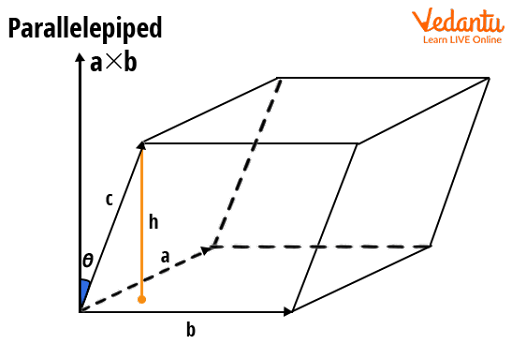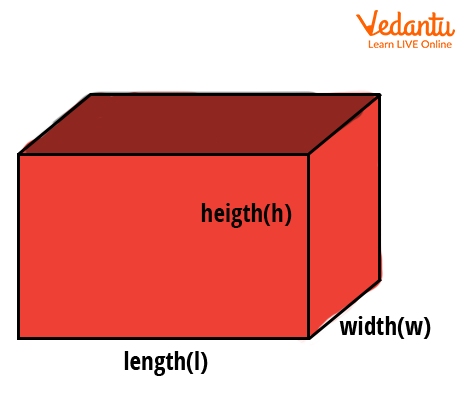Courses
Courses for Kids
Free study material
Free LIVE classes
More

# Volume of Parallelepiped - Properties and Formula based Solved Examples for JEE## Parallelepiped: A 3-D Shape with Parallelogram Faces

Last updated date: 19th Mar 2023
Total views: 80.4k
Views today: 1.59k

Parallelepiped is a 3-D shape with parallelogram faces. It is derived from a Greek word that means ‘an object with parallel planes’. It is made up of six parallelogram sides that combine to form a three-dimensional figure or a prism with a parallelogram base. A parallelepiped is a special case of the cube, cuboid and rhomboid. A cube is a parallelepiped with square sides on all sides. A cuboid and a rhomboid are both parallelepipeds with rectangle and rhombus-shaped faces. A parallelepiped is depicted in the figure below, with 'a', 'b', and 'c' as side lengths and 'h' as the parallelepiped's height.Image: Parallelepiped diagram

### Properties of Parallelepiped

Certain characteristics of a parallelepiped help us distinguish it from other 3-D shapes. These features are listed below.Image: Parallelepiped diagram showing one vertex of a parallelepiped at H

• Parallelepiped is a solid three-dimensional shape.

• There are eight vertices of a parallelepiped, twelve edges and six faces.

• A parallelepiped has all of its faces in the shape of a parallelogram.

• It has 12 face diagonals in total. It has two diagonals on each face.

• The diagonal that connects the vertices that are not on the same face is called the ‘body’ or ‘space diagonal’ of a parallelepiped.

• A parallelepiped is a prism with a parallelogram-shaped base.

• A parallelepiped's faces are mirror images of each other.

## Formula for Surface Area of parallelepiped

Surface area is defined as the total area covered by all of its surfaces.  There are two types of parallelepiped surface areas:

• Lateral Surface Area

• Total Surface Area

### 1. Parallelepiped Lateral Surface Area (LSA):

The lateral surface area of a parallelepiped is defined as the area of the parallelepiped side faces.

To compute the Lateral Surface Area

To calculate the LSA of a parallelepiped, add the areas covered by the four side faces.

### 2. Parallelepiped Total Surface Area (TSA):

It is defined as the area of all its faces. To calculate the TSA of a parallelepiped, add the areas covered by the six faces.

## Formula of Parallelepiped Surface Area:

The lateral surface area and total surface area of a parallelepiped are calculated as follows:

LSA of Parallelepiped = P $\times$ H.

TSA of Parallelepiped = LSA + 2$\times$ B = (P$\times$ H) + (2 $\times$B).

where B = Base area,

H = Parallelepiped height,

P = Base Perimeter.

## Volume of Parallelepiped Formula

If S is the area of the bottom face and H is the height of a parallelepiped, then the volume formula is

V = S$\times$H.

As the base of a parallelepiped is shaped like a parallelogram, we can use the formula for the area of a parallelogram to find the base area.

Parallelogram Area = Length$\times$Height.

## Rectangular Parallelepiped

When all six faces of a parallelepiped are rectangular, it is called a ‘rectangular parallelepiped’. It is a box-shaped structure in three dimensions. All of the parallel edges here are of the same length. The prism's base in this case is rectangular. The shoe box, which has a rectangular shape, is a real-life example of the same.

A rectangular parallelepiped is also called a ‘six-faced polyhedron’, also known as a ‘hexahedron’, each of which is a parallelogram.

A hexahedron has three parallel faces and a prism with a parallelogram base.Image:  Rectangular parallelepiped diagram

## Rectangular Parallelepiped Properties

• A three-dimensional solid figure is a rectangular parallelepiped. Furthermore, any of its three faces can be seen at the same time.

• It is made up of three sets of four parallel edges, each with equal length measurements.

• The diagonal of each face is referred to as the face diagonal.

• From the outside, each face appears to be a mirror image of the opposite face.

• Its shape resembles a prism with a parallelogram base. It was previously known as a six-faced polyhedron.

• A hexahedron is also formed by three pairs of parallel faces.

## Formula for Surface Area of Rectangular Parallelepiped

According to the text above, the rectangular parallelepiped is a three-dimensional box shape.

As the base of the parallelepiped in the rectangular parallelepiped shape is in the shape of a parallelogram, the rectangular parallelepiped base area formula is the area of the parallelogram, which is:

Rectangular Parallelepiped Base Area = length $\times$ height = a $\times$ b

Also, the lateral area is the product of the perimeter of the above-mentioned shape's base and the height of the six parallelograms faced prism, which is given as:

LSA = Perimeter of the rectangular parallelepiped's base $\times$ Height (h)

The total surface area is the sum of the LSA and twice the base area. We can write the statement mathematically as:

TSA = LSA + 2 $\times$ base area

## Formula for Volume of Rectangular Parallelepiped

For a Rectangular Parallelepiped having length ‘l,’ width ‘w,’ and a height ‘h,’

the rectangular volume formula is:

V  =  l $\times$ w $\times$ hImage:  Rectangular parallelepiped

The above formula is also called the ‘rectangular parallelepiped volume formula’.

Similarly, the rectangular parallelepiped volume formula  can be written in the following manner:

V  =  S $\times$ H

Here,

The area of the bottom face is denoted by S, and

H is the rectangular parallelepiped height.

## Conclusion

Parallelepiped is a 3-D shape with parallelogram faces. It is made up of six parallelogram sides that combine to form a three-dimensional figure or a Prism with a parallelogram base. To summarise, the rectangular parallelepiped in its specific form has six parallelograms as faces. This means that each parallelepiped has a unique connection to the tetrahedron and vice versa. This is because any pair of opposite tetrahedron edges specifically describes two parallel planes, one by each of the edges.

### Solved Examples on Parallelepiped

1. If the base face of a parallelepiped has opposite sides measuring 5 inches and 10 inches and its height is 7 inches, find the lateral surface area of the parallelepiped.

Solution:

Using the lateral area of the parallelepiped formula,
LSA = Perimeter of base $\times$ height.
LSA = 2$\times$ (5 + 10) $\times$ 7
= 224 inch2

2. A gift is packed in a rectangular box of dimensions 8 inches, 7 inches and 8 inches and it needs to be wrapped with gift paper. How much gift paper is required to wrap the gift box?

Solution:

The dimensions of the given gift box are,

length (l) = 10 in, width (w) = 7 in, height (h) = 8 in.

To find the amount of gift paper required, we need to find the total surface area of the box. Since the shape of the box can be compared to a rectangular parallelepiped,

\begin{align} \mathrm{TSA} &=2(\mathrm{lw}+\mathrm{wh}+\mathrm{hl}) \\ &=2(10 \times 7+7 \times 8+8 \times 10) \\ &=2(70+56+80)=412 \mathrm{in}^{2} \end{align}

## FAQs on Volume of Parallelepiped - Properties and Formula based Solved Examples for JEE

1. Which form of the parallelepiped resembles the 2-D shape?

The shape of the parallelepiped will resemble the parallelogram as the shape parallelepiped itself can be defined as a 3-D shape as all the faces are parallelograms. A parallelogram is made up of two opposite equal angles and two parallel lines.  A parallelepiped is a three-dimensional figure formed by six parallelograms, as stated above (the term ‘ rhomboid’ is also used sometimes with this same meaning). We can relate it to a parallelogram just as we relate a cube to a square.

2. When is an ordinary parallelepiped referred to as a rectangular parallelepiped?

An ordinary parallelepiped is a 3-D shape where all faces are parallelograms whereas the rectangular parallelepiped has all the faces in a rectangular shape. A parallelepiped will behave like a rectangular parallelepiped when all six of its faces resemble a rectangle. It is a box-shaped structure in three dimensions.  We can say that when all of the parallel edges of the parallelepiped have the same length, an ordinary parallelepiped is said to be the same as a rectangular parallelepiped.